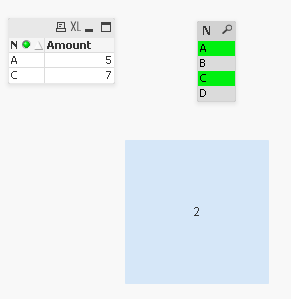# Groupe des Utilisateurs Francophones

cancel
Showing results for
Did you mean:Creator

## PROBLEM OF DIFFERENCE OF SUM

i have a problem and i need help

i'm a debutant

i have a dimension that contain products for exemple A B C D E F

i want to see the difference between the two products that he selected

for exemple if he selected A B

sum(A) - sum(b)

if he selected A C

sum(A) - sum(C)

thanks for help1 Solution

Accepted SolutionsMaster III
5 RepliesMaster III

Text box expression

=sum(if(Name=textbetween(GetFieldSelections(Name,',')&',',',',','),Amount))-sum(if(Name=textbetween(','&GetFieldSelections(Name,','),',',','),Amount))

Script

[

ID,Name, Amount

1,A,5

2,B,6

3,C,7

4,D,8

];Master III

FileMVP

maybe (in textbox)

=sum(if(Product=SubField(GetFieldSelections(Product,','),','), Amount))

-

sum(if(Product=SubField(GetFieldSelections(Product,','),',', 1), Amount))Creator
Author

thanks a lotPartner

Hi,

You can also try this using Set Analysis.

=Sum({<Name={\$(=SubField(Concat(Name, '|'), '|', 2))}>} Amount) - Sum({<Name={\$(=SubField(Concat(Name, '|'), '|', 1))}>} Amount)

Regards,

Jagan.Tags